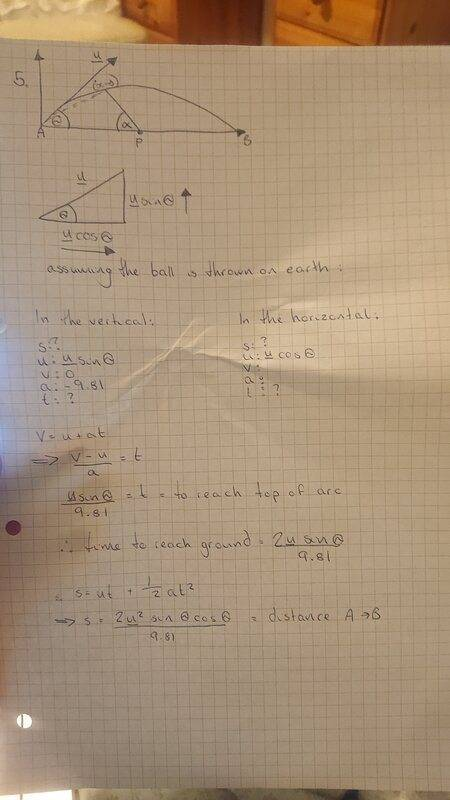# Launching a projectile to hit a target moving away from the launch point

Homework Statement:
A ball is launched at A with a speed u at an angle ϴ (from the horizontal). Show that if a point P moves so as to keep d/dt(tanα)=constant, then P will "catch" the ball at point B.
Relevant Equations:
tanϴ =tanα+tanβ## Answers and Replies

haruspex
Science Advisor
Homework Helper
Gold Member
2020 Award
Your attempt appears to be a random sequence of equations, not directed towards solving the problem.
First, find the x and y location of the projectile as a function of time. Don't substitute a value for g, just leave it as g.
Using those equations, find an equation for the position of P as a function of time.

Thanks for the help. I found the x and y location of the projectile to be x(t)=ut.cosϴ and y(t)=ut.sinϴ-1/2gt^2 but I'm unsure as to how to find an equation for P using this.

haruspex
Science Advisor
Homework Helper
Gold Member
2020 Award
Thanks for the help. I found the x and y location of the projectile to be x(t)=ut.cosϴ and y(t)=ut.sinϴ-1/2gt^2 but I'm unsure as to how to find an equation for P using this.
Just a bit of trig. What is the height of (x,y) above P? So what is the horizontal distance from (x,y) to P?